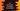# C# program to print the result of the division of two numbers## C# program to print the result of dividing two numbers:

Division is one of the basic arithmetic operations. It is also known as the number of times one number is contained in another number. / is known as the division operator. 10/2 is equal to 5. It means, we can divide 10 into 5 small parts of size 2.

11/2 will give a remainder of 1. We can divide 11 into 5 small parts of size 2 with a remainder 1. It is also equal to 5.5. 5 is called quotient.

We can use the same operator / to find the result of dividing two numbers. In this post, we will learn how to find the result of the division of two numbers and how to find the division by taking these numbers as inputs from the user.

### C# program to find the result of the division of two numbers:

Let’s take a look at the below program:

``````using System;

namespace Program
{
class Program
{
static void Main(string[] args)
{
Console.WriteLine(10 / 2);
Console.WriteLine(10 / 3);
Console.WriteLine(10 / 5);
Console.WriteLine(5 / 2);
Console.WriteLine(101 / 21);
}
}
}``````

It will print:

``````5
3
2
2
4``````

As you can see, it is printing the quotient values. If you want to print the exact values, you have to convert these values to double.

``````using System;

namespace Program
{
class Program
{
static void Main(string[] args)
{
Console.WriteLine((double)10 / 2);
Console.WriteLine((double)10 / 3);
Console.WriteLine((double)10 / 5);
Console.WriteLine((double)5 / 2);
Console.WriteLine((double)101 / 21);
}
}
}``````

It will print:

``````5
3.3333333333333335
2
2.5
4.809523809523809``````

### C# program to print the division result with user-input values:

We can also take the numbers as inputs from the user to calculate the division. Let’s take a look at the below program:

``````using System;

namespace Program
{
class Program
{
static void Main(string[] args)
{
int first, second;
float result;

Console.WriteLine("Enter the first number: ");

Console.WriteLine("Enter the second number: ");

result = (float)first / second;

Console.WriteLine(first + "/" + second + " = " + result);
}
}
}``````

This program reads the numbers and stores these in first and second integer variables. The result is converted to float.

It will print output as below:

``````Enter the first number:
100
Enter the second number:
2
100/2: 50

Enter the first number:
100
Enter the second number:
3
100/3 = 33.333332``````

### How to find the quotient and remainder:

As you have seen in the first example above, the division operator returns the quotient value. If you want to find the remainder of a division, you can use the modulus operator or % operator. a % b returns the remainder of a/b.

Let’s change the above program to print quotient and remainder:

``````using System;

namespace Program
{
class Program
{
static void Main(string[] args)
{
int first, second, quotient, remainder;

Console.WriteLine("Enter the first number: ");

Console.WriteLine("Enter the second number: ");

quotient = first / second;
remainder = first % second;

Console.WriteLine(first + "/" + second + " = " + "quotient = " + quotient + ", remainder = " + remainder);
}
}
}``````

It will print output as below:

``````Enter the first number:
100
Enter the second number:
3
100/3 = quotient = 33, remainder = 1

Enter the first number:
222
Enter the second number:
11
222/11 = quotient = 20, remainder = 2

Enter the first number:
121
Enter the second number:
11
121/11 = quotient = 11, remainder = 0``````